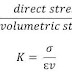Hello friends.
Today we are going to learn about some important definition used in the study of SOM like bulk modulus of elasticity, modulus of rigidity and Poisson’s ratio. In our previous articles, we have learned about strainstress and their types and relationship. We have also discussed about young modulus of elasticity in my last post. This article gives you brief information about the other three constants.
Let’s start the discussion.

# Elastic Constant:

### Bulk Modulus of Elasticity:

The ratio of direct stress to linear strain under the elastic limit is known as the young modulus of elasticity. It is a one-dimensional constant which gives information about the change in linear dimension under loading condition. But when a body undergoes any force, both its linear as well as lateral dimensions will change accordingly. This will change the overall dimension or volume of the object.
The change in volume to its original volume under loading condition is known as volumetric strain and the ratio of direct stress to volumetric strain is known as the bulk modulus of elasticity. It is defined by symbol K.

### Modulus of Rigidity:

When we applied a shear load (parallel to the object) on an object, the linear dimensions of the objects remain the same but the shape of the body deforms. The strain associated with the shear stress is known as shear strain. The ratio of shear stress to shear strain is known as modulus of Rigidity. This constant is represented by symbol G.

### Poisson’s Ratio:

It is another most useful constant in the study of the strength of the material. We have discussed about lateral strain and linear stain in our previous article.
The ratio of change in dimension to original dimension in direction of applied force is known as linear strain or longitudinal strain and the ratio of change in dimension to original dimension in a direction perpendicular to the applied force is known as lateral strain.

The ratio of lateral strain to linear strain is known as Poisson’s Ratio. It is represented by μ.

This is a basic introduction of bulk modulus of elasticity, modulus of rigidity and Poisson’s Ratio. If you have any query regarding this article, ask by commenting. Subscribe to our website for more articles regarding the strength of the material. Thanks for reading it.
Elastic Constant : Bulk Modulus, Modulus of Rigidity and Poisson’s RatioReviewed by Admin on July 27, 2018 Rating: 5